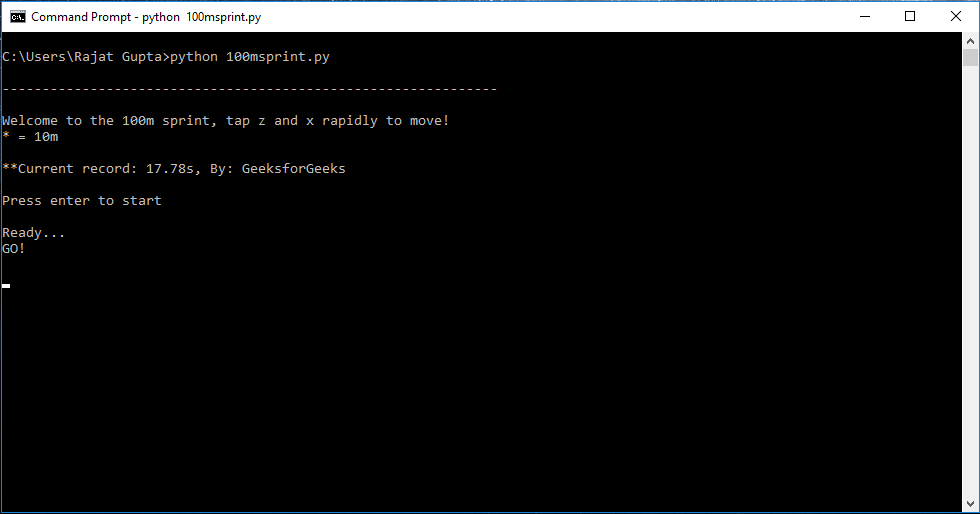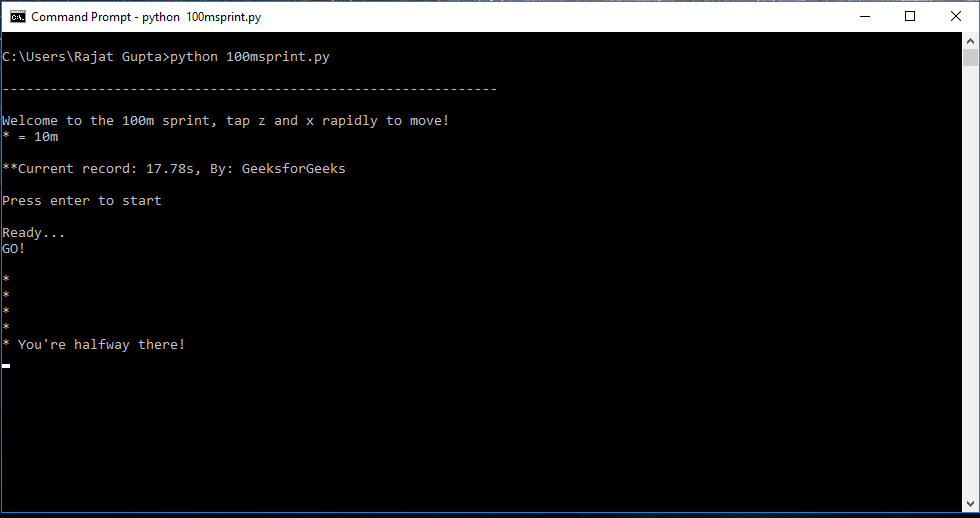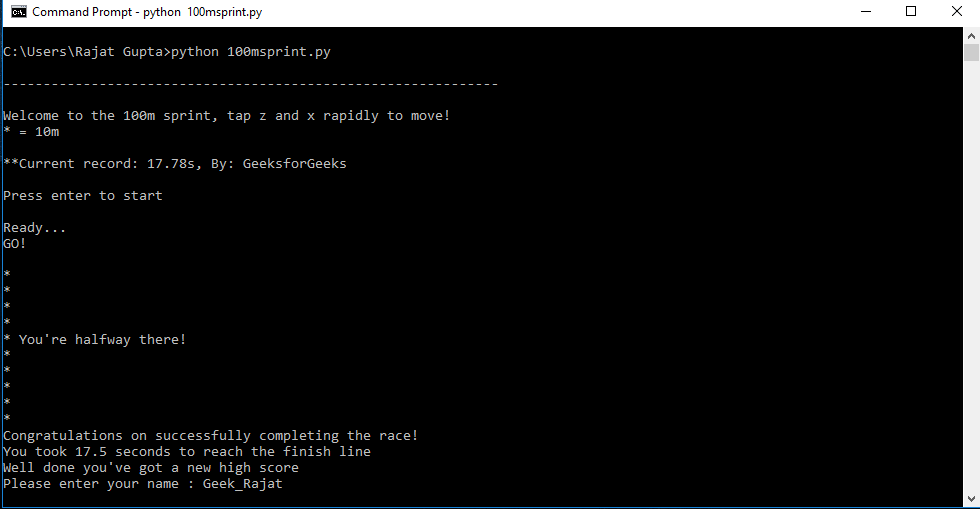# Simple Keyboard Racing with Python

Let’s make a simple keyboard racing game using Python. In the game, the participant clicks a pair of keys in quick succession and the program shows the total time taken by the racer to cover the distance.

Rules:
As soon as you see ‘GO!’ on screen, start pressing the keys ‘z’ and ‘x’. A ‘*’ sign is shown for every metre covered. Pressing ‘z’ and ‘x’ once will be counted as 1 metre; targets is to cover 10 metres.

Modules Used:

```msvcrt : Used to get keystroke as input for race
time : Used to calculate time taken to complete the race
```

Note that MSVCRT module can only function on a terminal window, not on a GUI program/IDE.

Below is the code:

 `import` `msvcrt ` `import` `time ` ` `  `high_score ``=` `17.78` `name ``=` `"GeeksforGeeks"` `while` `True``: ` `    ``distance ``=` `int``(``0``) ` `    ``print``(``'\n--------------------------------------------------------------'``) ` `    ``print``(``'\n\nWelcome to the 100m sprint, tap z and x rapidly to move!'``) ` `    ``print``(``'* = 10m'``) ` `    ``print``(``'\nCurrent record:'` `+` `str``(high_score) ``+` `' by: '` `+` `name) ` `    ``print``(``'\nPress enter to start'``) ` `    ``input``() ` `    ``print``(``'Ready...'``) ` `    ``time.sleep(``1``) ` `    ``print``(``'GO!'``) ` ` `  `    ``start_time ``=` `time.time() ` `    ``while` `distance < ``10``: ` ` `  `        ``k1 ``=` `msvcrt.getch().decode(``'ASCII'``) ` `        ``if` `k1 ``=``=` `'z'``: ` `            ``k2 ``=` `msvcrt.getch().decode(``'ASCII'``) ` `            ``if` `k2 ``=``=` `'x'``: ` `                ``distance ``+``=` `1` `                ``if` `distance ``=``=` `5``: ` `                    ``print``(``"* You're halfway there!"``) ` `                ``elif` `distance ``%` `1` `=``=` `0``: ` `                    ``print``(``'*'``) ` ` `  `    ``fin_time ``=` `time.time() ``-` `start_time ` `    ``fin_time ``=` `round``(fin_time, ``2``) ` ` `  `    ``print``(``'Congratulations on successfully completing the race!'``) ` `    ``print``(``'You took'``, fin_time, ``'seconds to reach the finish line'``) ` ` `  `    ``if` `fin_time < high_score: ` `        ``print``(``"Well done you've got a new high score "``) ` `        ``name ``=` `input``(``"Please enter your name : "``) ` `        ``high_score ``=` `fin_time `

Output:Game InitiateGame in ProgressGame Finished: New High Score

My Personal Notes arrow_drop_upCheck out this Author's contributed articles.

If you like GeeksforGeeks and would like to contribute, you can also write an article using contribute.geeksforgeeks.org or mail your article to contribute@geeksforgeeks.org. See your article appearing on the GeeksforGeeks main page and help other Geeks.

Please Improve this article if you find anything incorrect by clicking on the "Improve Article" button below.

Article Tags :

1

Please write to us at contribute@geeksforgeeks.org to report any issue with the above content.